# Quantitative Python

Risk management with Python

By Rafael Ballestas | April 9, 2019 | Category: Philosophy

Now that we have an understanding of risk concepts such as the loss exceedance curve, value-at-risk, Bayes Rule, and fitting distributions, we would like to have a realiable, extensible and preferably open tool to perform these computations. In the background, we have used a spreadsheet, which is hard to extend. We have used GNU Octave, which is good, but not a proper programming language. Our favorite language at Fluid Attacks, `Python`, has modules for statistics, scientific computation and even finance itself. Let’s take it for a spin around this risky neighborhood.

`Python` has a whole ecosystem for numerical computing (v.g. `Numpy`) and data analysis (`Pandas`) and is well on its way to becoming a standard in Open Science. Being a free and open source tool, there are also many derived projects which make life easier when coding, such as the `Jupyter` Notebook, which allows us to selectively run code snippets, much like in commercial packages such as `Matlab` and `Mathematica`. This enables and encourages, at least, initial exploration, although it might not be the best fit for developing more involved code.

Let us see how we can automate the generation of a loss exceedance curve (`LEC`) via Monte Carlo simulation. Here we will closely follow our article on the subject, so as not to duplicate information. In that article, we wanted to find a distribution for losses based on expert estimations of occurrence likelihood and confidence intervals for the impact: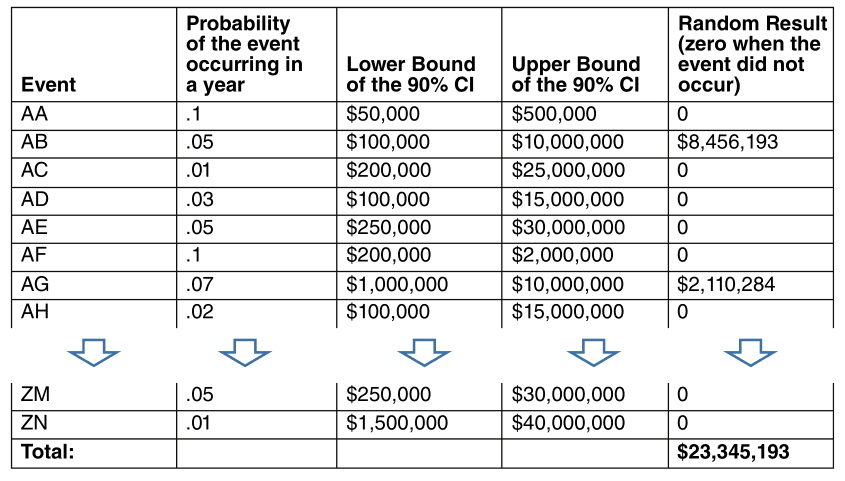Figure 1. Table with input data.

So we need to read those values in our script. Since this is tabular information of the kind that would be useful to view, say, in a spreadsheet, it will be convenient to read this into a `Pandas` dataframe:

Importing pandas and reading data.

``````import pandas as pd
``````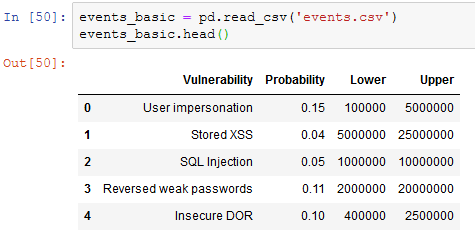Figure 2. Data as imported into Jupyter like in our Monte Carlo article.

We declare an event happens if a number taken at random is beyond a certain threshold, given by the second column in the table above:

``````def event_happens(occurrence_probability):
return np.random.rand() < occurrence_probability
``````

If and when the event happens, we next need to know the extent of the loss due to this single event. Recall that we modeled this with a lognormal variable, whose parameters we got from the estimated confidence interval:

``````def lognormal_event_result(lower, upper):
mean = (np.log(upper) + np.log(lower))/2.0
stdv = (np.log(upper) - np.log(lower))/3.29
return np.random.lognormal(mean, stdv)
``````

All of the events in the above table can happen in a single year, so to simulate a scenario, we need to find out, for each of them, if they happen, and how much money they will cost us. Finally, we add all the losses and return that single number as a summary of the losses in a simulated year:

``````def simulate_scenario(events):
total_loss = 0
for _, event in events.iterrows():
if event_happens(event['Probability']):
total_loss += lognormal_event_result(event['Lower'],event['Upper'])
``````

Now, the crucial step in Monte-Carlo simulation is to simulate many scenarios and record those results. Let us write a function that does just this, returning the results in a basic `Python` list, which we could later turn, if we so wished, into a `Pandas` or `Numpy`-native structure for statistical analysis. The function takes as input the number of times we want to simulate scenarios:

``````def monte_carlo(events, rounds):
list_losses = []
for i in range(rounds):
loss_result = simulate_scenario(events)
list_losses.append(loss_result)
return list_losses
``````

## Going graphic

Just to get a feeling for the results, let us run a thousand scenarios and plot them, that is, the result of each simulated year, in the order in which they were obtained. As foretold, we could convert the results into a `Pandas` `DataSeries`, if anything, to illustrate how they work. We also need to import `Matplotlib` for visualization:

``````import matplotlib.pyplot as plt
results = monte_carlo(events_basic, 1000)
results_series = pd.Series(results)
results_series.plot()
``````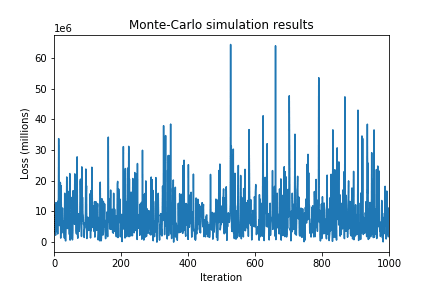Figure 3. Raw Monte-Carlo results

It can be observed that the vast majority of them are in the fringe between 0 and 15 million. But it is not infeasible to have results that are way beyond the central interval. In order to rule out what’s simple chance and what is really happening due to the distribution of loss, we can simply run more scenarios. Tens or hundreds of thousands of scenarios is a good rule of thumb, without sacrificing performance. A thousand runs takes around 5 seconds, and 10000 takes around 50. At some point adding more simulations does not necessarily improve the quality of results. Your mileage may vary.

No matter the number of scenarios, the results are not as useful as they could be until we aggregate them, v.g., in a histogram. `Pandas` also provides a shorthand for this:

``````results_series.hist(bins = 15)
``````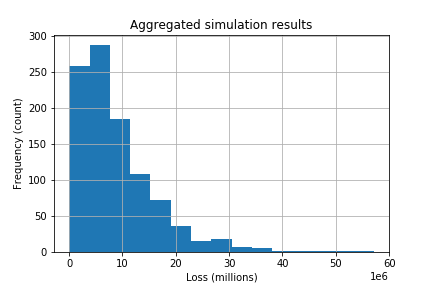Figure 4. Histogram of results

We’re getting closer to the loss exceedance curve, but not there yet. We can estimate probabilities simply by counting occurrences and normalizing by dividing by the number of rounds and multiplying by 100. Hence the estimated "probability" of a single value is the normalized number of times that value appeared in the simulation. So let us take evenly spaced values, and count the number of times each of those values is exceeded (or matched). The `numpy` function `cumsum` does just that, except in the opposite order: it adds the values seen up to a moment. So if we take the intervals and the counts separately, revert the counts list and then do `cumsum` on it, we get what we need, in reverse order. To fix that we simply revert again:

``````import numpy as np
result_nparray = np.array(results_list)
hist, edges = np.histogram(results_nparray, bins = 40)
cumrev = np.cumsum(hist[::-1])[::-1]*100/len(results_nparray)
plt.plot(edges[:-1], cumrev)
``````

And voilà, we get our loss exceedance curve as we sought: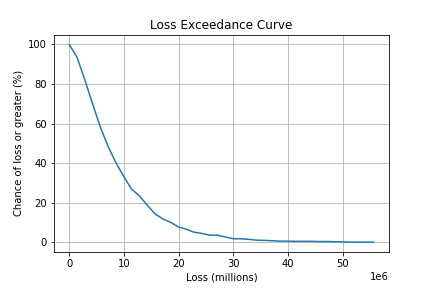Figure 5. Simple loss exceedance curve like in our Monte Carlo article.

We can repeat the procedure with a more moderate dataset to obtain the inherent risk `LEC`, in the sense that the probabilities and impact CIs are lower. And finally, to obtain the risk tolerance curve, we give a few points obtained from interviewing someone in charge, as described in the original article and fitting a curve to it using SciPy’s Interpolation functions:

``````from scipy import interpolate
xs = np.array([1,2,3,7,9])*(1e6)
tols = np.array([100,60,10,2,1])
xint = np.linspace(min(xs), max(xs))
fit = interpolate.interp1d(xs, tols, kind='slinear')
plt.plot(xint, fit(xint))
``````

All together in a single plot: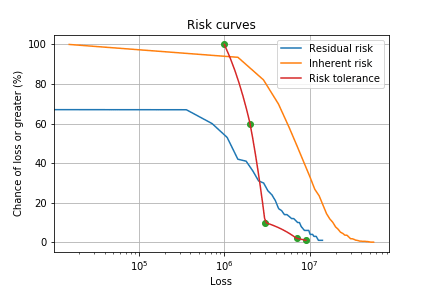Figure 6. Loss exceedance curves like in our Monte Carlo article.

## Risk measures

Now obtaining the 5% value at risk is simply a matter of asking for the 95th percentile of the "distribution", i.e., the actual simulation results, in its `Numpy`-array incarnation:

``````>>> np.percentile(results_nparray, 95)
23360441.53826834
``````

Hence the `VaR`, according to this particular simulation is a little over \$23 million. It is just as simple to obtain the tail value at risk. If we had a mathematical function for the distribution we would have to compute an integral in order to obtain it, but since what we have is a discrete approximation to it, i.e., a simple table of values, we can just average the values that are under the `VaR`:

``````>>> np.average(results_nparray[results_nparray >= var])
31949559.99328234
``````

Thus in case of a `VaR` breach, we can expect the loss to be of little less than \$32 million.

Let us simulate the input values for the simulation, as if we were running the simulation every day with different occurrence probabilities and impacts. Let us make up a `DataFrame` with random values for the inputs:

``````def gen_random_events():
probability_column = np.random.random_sample(30)*0.1
lower_ci_column    = np.random.random_sample(30)*(1e6)
upper_ci_column    = np.random.random_sample(30)*(9e6)+1e6
dicc = {'Probability' : probability_column,
'Lower' : lower_ci_column,
'Upper': upper_ci_column}
events_rand = pd.DataFrame(dicc)
return events_rand
``````

Next we run Monte-Carlo on those, once for each day of a fictitious month, compute the `VaR` and `tVaR` for each day and observe how they evolve: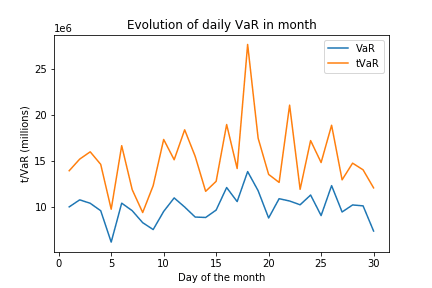Figure 7. Fabricated VaR monitoring example like in our VaR article.

Since this was a made-up example and the probabilities are sampled simply, i.e., from a uniform distribution, the results are, well, uniform. However for the sake of conclusion, we can imagine there is a steady, if slow, trend towards raising the `VaR`. It is interesting that the highest peak in `tVaR` corresponds to a `VaR` that is not that different from its neighbors. This goes to show that one is not just a simple function of the other, which is often the case in dealing with uncertainty.

## References

1. C. Davidson-Pilon (2019). Probabilistic Programming and Bayesian Methods for Hackers.

2. C. Motiff (2019). Monte Carlo Simulation with Python

3. B. Mikulski (2018). Monte Carlo simulation in Python

## Appendix: Full script

Download Python script or as Jupyter notebook and input data for inherent risk and residual risk.

Ready to try Continuous Hacking?

Discover the benefits of our comprehensive Continuous Hacking solution, which hundreds of organizations are already enjoying.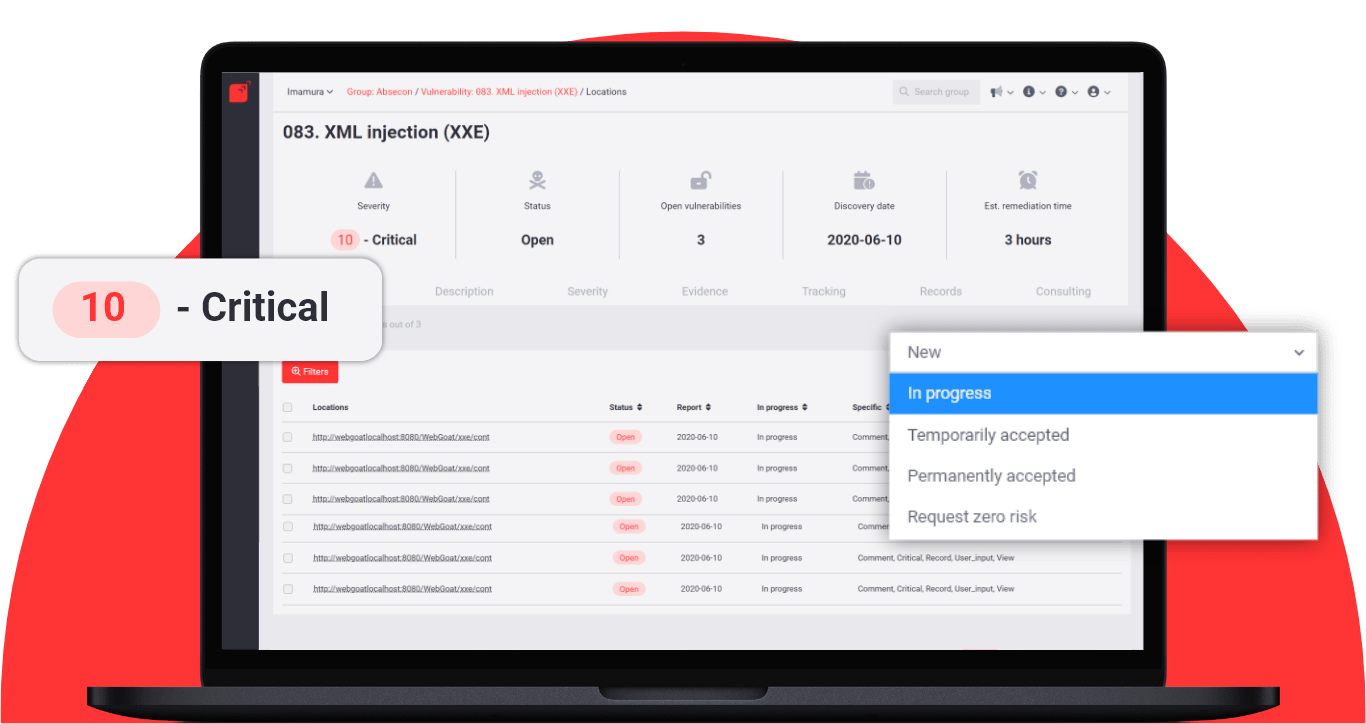Start free trial